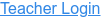### Description

Hang masses from springs and adjust the spring constant and damping. Transport the lab to different planets, or slow down time. Observe the forces and energy in the system in real-time, and measure the period using the stopwatch.

### Sample Learning Goals

• Determine the factors which affect the period of oscillation
• Find the value of g on Planet X
• Design an experiment to determine the mass of an unknown object
• Describe the relationship between the velocity and acceleration vectors, and their relationship to motion, at various points in the oscillation
• Explain how the free-body diagram of the mass changes throughout its oscillation
• Explain the Conservation of Mechanical Energy using kinetic, elastic potential, gravitational potential, and thermal energy
Version 1.0.10